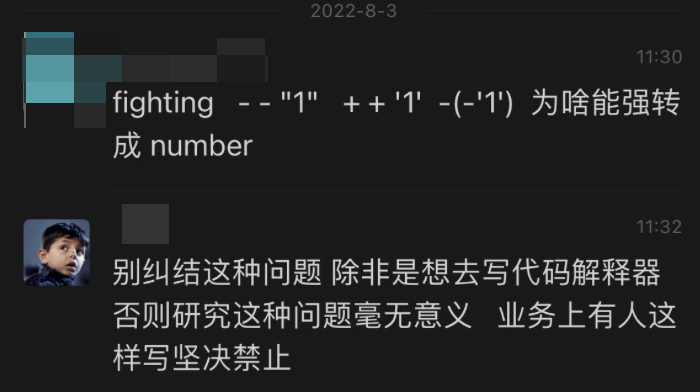# 写让人看得懂的代码``````// 交换两数，结果 a: 15 b: 10
let a = 10
let b = 15
a ^= b
b ^= a
a ^= b

// 判断存在子串
if (~"hello".indexOf("h")) {
// do something
}

// 交换 0 1
let a = 1;
// in some loop
{
a ^= 1;
console.log(a); // 0,1,0,1,0 ...
}

// 嵌套三目运算符或逻辑运算符
const a = condition1 ? 1 : condition2 ? 2 : 3;
const b = x || y && c && d || e;

// ...
``````

### 代码是写给人看的

* 你是否被数月前甚至数星期前自己写的代码困扰过
* 你的同事是否经常跑来问你某个逻辑之前为什么要那样实现

### 代码的意义应该不言自明

``````let result;
if (isA()) {
result = doSomthingA()
} else if (isB()) {
result = doSomethingB()
} else {
result = doSomethingC()
}
``````

``````let result = isA() ? doSomthingA() : isB() ? doSomethingB() : doSomethingC()
``````

* 改别人的代码
* 别人改我的代码
* review 别人的代码
* 别人 review 我的代码
* …